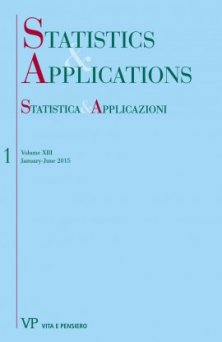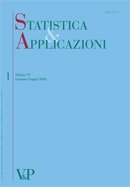Your browser does not support JavaScript!

Z.R. Al-Rawi

Z.R. Al-Rawi è un docente presso il dipartimento di Statistica dell'Università di Irbid a Yarkmouk in Giordania.
Share:

Author's books

A Generalized multivariate skew-normal distribution with applications to spatial and regression predictions digitalformat:Article| STATISTICA & APPLICAZIONI - 2015 - 1
Year: 2015
In this paper, a generalization to the multivariate skew-normal distribution of Arnold and Beaver (2002) is proposed. Also several distributional properties of the proposed distribution are explored. The proposed distribution has been used to define a stochastic process called the generalized-skew Gaussian process...
Comparison of several confidence intervals for normal distribution with known coefficient of variation
Free
digitalformat:Article| STATISTICA & APPLICAZIONI - 2010 - 1
Year: 2010
In this paper, we consider the problem of constructing confidence intervals for the normal population mean when the coefficient of variation is known. We obtain 13 confidence intervals using different pivots. Also we conduct a simulation study to compare the expectations as well as the standard deviations of the intervals lengths. Keywords: Coefficient of Variation, Normal Distribution, Pivotal Method, Confidence Interval.